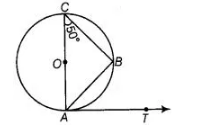# In figure, AB is a chord of the circle and AOC

Question:

In figure, AB is a chord of the circle and AOC is its diameter such that ∠ACB = 50°. If AT is the tangent to the circle at the point A, then ∠BAT is equal to(a) 45°

(b) 60°

(c) 50°

(d) 55°

Solution:

(c) In figure, AOC is a diameter of the circle. We know that, diameter subtends an angle 90° at the circle.

So, $\angle A B C=90^{\circ}$

In $\triangle A C B, \quad \angle A+\angle B+\angle C=180^{\circ}$ [since, sum of all angles of a triangle is $180^{\circ}$ ]

$\Rightarrow \quad \angle A+90^{\circ}+50^{\circ}=180^{\circ}$

$\Rightarrow$$\angle A+140=180$

$\angle A=180^{\circ}-140^{\circ}=40^{\circ}$

$\angle A$ or $\angle O A B=40^{\circ}$

Now, $A T$ is the tangent to the circle at point $A$. So, $O A$ is perpendicular to $A T$.

$\therefore$ $\angle O A T=90^{\circ}$ [from figure]

$\Rightarrow$ $\angle O A B+\angle B A T=90^{\circ}$

On putting $\angle O A B=40^{\circ}$, we get

$\Rightarrow \quad \angle B A T=90^{\circ}-40^{\circ}=50^{\circ}$

Hence, the value of $\angle B A T$ is $50^{\circ}$.